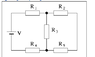# Equivalent resistance of a circuit

EchoTheCat

## Homework Statement

Consider the electric circuit shown in the figure. Assume that V = 13.7 V, R1 = R2 = R3 = R4 = R5 = 7.00 Ω. What is the equivalent resistance of the circuit?

## Homework Equations

total voltage of a series circuit is the sum of the voltage of all the resistors
total current of a series circuit is the same as the current through any individual resistor
total resistance of a series circuit is the sum of the resistance of all the resistors

total voltage of a parallel circuit is the same as the voltage through any individual resistor
total current of a parallel circuit is the sum of the current of all of the resistors
the inverse of the total resistance of a parallel circuit is the sum of the inverse of each resistance

## The Attempt at a Solution

I guess I'm confused as to how to 'combine' resistors to turn this into just a series circuit? I see both parallel elements and series elements.

EchoTheCat
Here's the picture.

I did try considering the circuit as a parallel circuit R1, R3, and R4 together with total resistance 21 and R2 and R5 together with total resistance 14 but that didn't work

#### Attachments

•circuit.PNG
1.4 KB · Views: 373
Mentor
In this circuit, it is easiest to start at the tight and fold up the resistances right-to-left.

What 2 resistors are in series on the right side? What is their combined resistance? Then what other resistor is in parallel with that combined resistance? Combine those parallel resistances. Then finally, what 3 resistances are now in series? Combine them to get the answer.

Can you try that and show us your math for each step? Thanks.EchoTheCat
Resistors 2 and 5 form a series circuit on the right side; their combined resistance is 14. This is parallel to Resistor 3; their combined resistance is 1/R = 1/7 + 1/14 --> R = 4.66667. The three resistors now in series are 1, 4, and combined resistors of 2, 3, and 5. The sum of the resistance is 4.66667 + 7 + 7 =18.66667 ohms.

And it works!
Thank you!

•berkeman